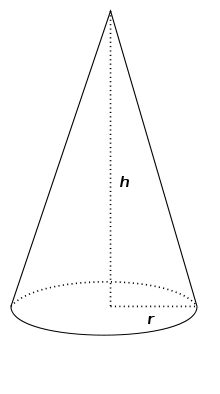# Rotating 7947

In the rotating cone = 100π
S rotating cone = 90π
v =?
r =?

r1 =  5
v1 =  12
r2 =  4.4721
v2 =  15

### Step-by-step explanation:Did you find an error or inaccuracy? Feel free to write us. Thank you!

Tips to related online calculators
Looking for help with calculating roots of a quadratic equation?
Do you have a system of equations and looking for calculator system of linear equations?
Tip: Our volume units converter will help you with the conversion of volume units.

#### You need to know the following knowledge to solve this word math problem:

We encourage you to watch this tutorial video on this math problem: